# User:Tohline/SSC/Structure/BiPolytropes/MurphyUVplane

(Difference between revisions)
 Revision as of 08:28, 2 May 2015 (view source)Tohline (Talk | contribs) (→Critique of Murphy's Model Characteristics: Add footnote to table of numbers)← Older edit Revision as of 11:59, 14 May 2015 (view source)Tohline (Talk | contribs) (→Critique of Murphy's Model Characteristics: Insert comparison table from another chapter)Newer edit → Line 492: Line 492:
NOTE: As is [[User:Tohline/SSC/Structure/BiPolytropes/Analytic1_5#Murphy.27s_Example_Model_Characteristics|explained in an accompanying discussion]], we suspect that the two numbers drawn from Murphy's Table 3 that are displayed here in a red font contain typographical errors. NOTE: As is [[User:Tohline/SSC/Structure/BiPolytropes/Analytic1_5#Murphy.27s_Example_Model_Characteristics|explained in an accompanying discussion]], we suspect that the two numbers drawn from Murphy's Table 3 that are displayed here in a red font contain typographical errors. +
+ + + + + +
+ +
+ Some Model Characteristics (assuming $\mu_e/\mu_c$ = 1) +
+ Model + + Specified + + Analytically Determined Here + + … and Constraint Implications +
+ $~\xi_i$ + + $~m$ + + $~\Delta_i$ + + $~(A_0\eta)_\mathrm{root}$ + + $~\frac{A_0}{3^{1/2}} \biggl( \frac{\mu_e}{\mu_c} \biggr)$ + + $~B_0$ + + $~\frac{B_0}{3^{1/4}} \biggl(\frac{\mu_e}{\mu_c}\biggr)^{1/2} \theta_i^{-1}$ + + $~\xi_s$ + + $~V_{1E}$ + + $~V_{5F}$ + + $\frac{(n_c+1)V_{1E}}{(n_e+1)V_{5F}}$ + + $~U_{1E}$ + + $\frac{U_{1E}}{U_{5F}}$ +
13.2678E-0201.154410.0614307.8945.779294.392091.73923.5598E-041.1866E-41.0002.99981.000
20.815401.242912.010114.7291.255671.0686536.3560.232120.0773721.0002.86441.000
31.659801.491819.758511.90411.012061.2814644.9841.148120.382711.0002.39951.000
42.091411.732831.996415.29900.875631.6039835.00162.199130.733041.0001.988941.000
52.730212.8749100.00$\pi$115.0650.124080.643704.65397.257792.419261.0001.027031.000
63.141526.28314191268$\pi$170.44223$e^{2\pi}$1.8966E-050.488623.1417783.3907E+041.1302E+041.0002.9106E-041.000
+ Corresponding Values Extracted Directly from [http://adsabs.harvard.edu/abs/1983PASAu...5..175M Murphy's (1983)] Table 3 + +   +
[[File:Table3CompleteCol1.png|center|50px|Column 1 from Table 3 of Murphy (1983)]][[File:Table3CompleteCol2.png|center|123px|Column 3 from Table 3 of Murphy (1983)]] [[File:Table3CompleteCol3.png|center|67px|Column 3 from Table 3 of Murphy (1983)]][[File:Table3CompleteCol4.png|center|117px|Column 4 from Table 3 of Murphy (1983)]] [[File:Table3CompleteCol5.png|center|71px|Column 5 from Table 3 of Murphy (1983)]][[File:Table3CompleteCol6.png|center|83px|Column 6 from Table 3 of Murphy (1983)]][[File:CommentButton02.png|left|100px|Comment by J. E. Tohline on 11 May 2015: + The heading on this last column of numbers extracted from Murphy's (1983) paper has a subscript "J" whereas the subscript should be "R" to indicate the configuration's total radius.]]
+ See an [[User:Tohline/SSC/Structure/BiPolytropes/MurphyUVplane#Chandrasekhar.27s_U_and_V_Functions|accompanying discussion]] for definitions of the functions, $~U_{1E}$, $~V_{1E}$, $~U_{5F}$, and $~V_{5F} \, .$
+ + + + + + + + + + + + + + + + + + + + + + + + + + + + + + + + + + + + + + + + + + + + + + + + + + + + + + + + + + + + + + + + + + + + + + + + + + + + + + + + + + + + + + + + + + + + + + + + + + + + + + + + + + + + + + + + + + + + + + + + + + + + + + + + + + + + + + + + + + + + + + + + +

# UV Plane Functions as Analyzed by Murphy (1983)

This chapter supports and expands upon an accompanying discussion of the construction of a bipolytrope in which the core has an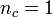$~n_c=1$ polytropic index and the envelope has an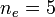$~n_e=5$ polytropic index. This system is particularly interesting because the entire structure can be described by closed-form, analytic expressions. Here we provide an in-depth analysis of the work published by J. O. Murphy (1983, Proc. Astr. Soc. of Australia, 5, 175) in which the derivation of this particular bipolytropic configuration was first attempted. As can be seen from the following list of "key references," however, this publication was only one of a series of interrelated works by Murphy. We will henceforth refer to this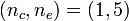$~(n_c, n_e) = (1, 5)$ system as "Murphy's bipolytrope."

## Relevant Lane-Emden Functions

As is detailed in our accompanying discussion, the Lane-Emden function governing the structure of the$~n_c = 1$ core of Murphy's bipolytrope is,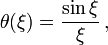$\theta(\xi) = \frac{\sin\xi}{\xi} \, ,$

and the first derivative of this function with respect to the dimensionless radial coordinate,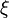$~\xi$, is,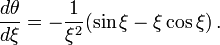$\frac{d\theta}{d\xi} = -\frac{1}{\xi^{2}} (\sin\xi - \xi\cos\xi) \, .$

Also as is detailed in our accompanying discussion, the Lane-Emden function governing the structure of the$~n_e = 5$ envelope of Murphy's bipolytrope is,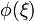$~\phi(\xi)$$~=$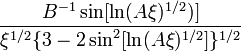$~\frac{B^{-1}\sin[\ln(A\xi)^{1/2})]}{\xi^{1/2}\{3-2\sin^2[\ln(A\xi)^{1/2}]\}^{1/2}}$$~=$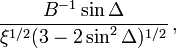$~\frac{B^{-1}\sin\Delta}{\xi^{1/2}(3-2\sin^2\Delta)^{1/2}} \, ,$

and the first derivative of this function is,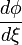$~\frac{d\phi}{d\xi}$$~=$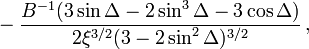$~- \frac{B^{-1}(3\sin\Delta - 2\sin^3\Delta -3\cos\Delta) }{2\xi^{3/2}(3-2\sin^2\Delta)^{3/2}} \, ,$

where we have adopted the shorthand notation,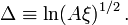$~\Delta \equiv \ln(A\xi)^{1/2} \, .$

## Chandrasekhar's U and V Functions

As presented by Murphy (1983), most of the development and analysis of this model was conducted within the framework of what is commonly referred to in the astrophysics community as the "U-V" plane. Specifically in the context of the model's$~n_c=1$ core, this pair of referenced functions is: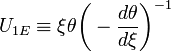$~U_{1E} \equiv \xi \theta \biggl(- \frac{d\theta}{d\xi}\biggr)^{-1}$$~=$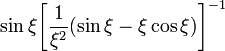$~\sin\xi\biggl[\frac{1}{\xi^{2}} (\sin\xi - \xi\cos\xi)\biggr]^{-1}$$~=$$~\frac{\xi^2}{(1 - \xi\cot\xi)} \, ;$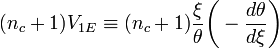$~(n_c+1) V_{1E} \equiv (n_c+1)\frac{\xi}{ \theta} \biggl(- \frac{d\theta}{d\xi}\biggr)$$~=$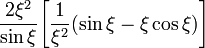$~\frac{2\xi^2}{\sin\xi} \biggl[ \frac{1}{\xi^{2}} (\sin\xi - \xi\cos\xi) \biggr]$$~=$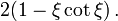$~ 2(1 - \xi\cot\xi) \, .$

Correspondingly, in the context of the model's$~n_e=5$ envelope, the pair of referenced functions is: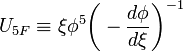$~U_{5F} \equiv \xi \phi^5 \biggl(- \frac{d\phi}{d\xi}\biggr)^{-1}$$~=$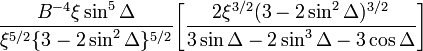$~ \frac{B^{-4}\xi \sin^5\Delta}{\xi^{5/2}\{3-2\sin^2\Delta\}^{5/2}} \biggl[ \frac{2\xi^{3/2}(3-2\sin^2\Delta)^{3/2}}{3\sin\Delta - 2\sin^3\Delta -3\cos\Delta } \biggr]$$~=$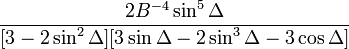$~ \frac{2B^{-4}\sin^5\Delta}{[3-2\sin^2\Delta][3\sin\Delta - 2\sin^3\Delta -3\cos\Delta ]}$$~=$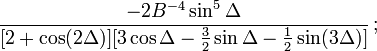$~ \frac{-2B^{-4}\sin^5\Delta}{[2+\cos(2\Delta)][3\cos\Delta - \frac{3}{2}\sin\Delta - \frac{1}{2}\sin(3\Delta) ]} \, ;$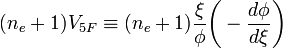$~(n_e+1) V_{5F} \equiv (n_e+1) \frac{\xi}{ \phi} \biggl(- \frac{d\phi}{d\xi}\biggr)$$~=$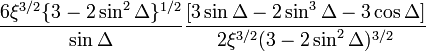$~\frac{6\xi^{3/2}\{3-2\sin^2\Delta\}^{1/2}} {\sin\Delta} \frac{[3\sin\Delta - 2\sin^3\Delta -3\cos\Delta ] }{2\xi^{3/2}(3-2\sin^2\Delta)^{3/2}}$$~=$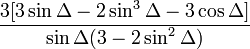$~\frac{3[3\sin\Delta - 2\sin^3\Delta -3\cos\Delta ] }{\sin\Delta (3-2\sin^2\Delta)}$$~=$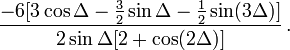$~\frac{-6[3\cos\Delta - \frac{3}{2}\sin\Delta - \frac{1}{2}\sin(3\Delta) ] }{2\sin\Delta [2+\cos(2\Delta)]} \, .$

In an effort to demonstrate correspondence with the published work of Murphy (1983), we have reproduced his expressions for these governing U-V functions in the following boxed-in image.

 U-V Functions Extracted from Murphy (1983) (slightly edited before reproduction as an image, here)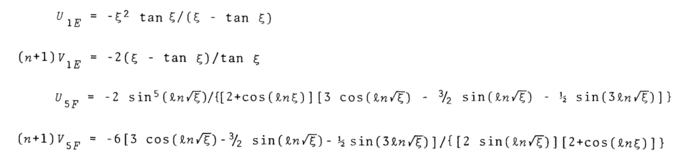The match between our expressions and those presented by Murphy becomes clear upon recognizing that, in our notation,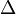$~\Delta$$~=$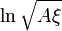$~\ln\sqrt{A\xi}$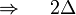$~\Rightarrow ~~~~ 2\Delta$$~=$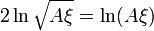$~2 \ln\sqrt{A\xi} = \ln(A\xi)$ and,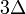$~3\Delta$$~=$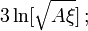$~3\ln[\sqrt{A\xi}] \, ;$

and, in laying out these function definitions, Murphy has implicitly assumed that the two scaling coefficients,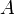$~A$ and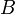$~B$, are unity.

CAUTION: Presented in this fashion — that is, by using$~\xi$ to represent the dimensionless radial coordinate in all four expressions — Murphy's expressions seem to imply that the independent variable defining the radial coordinate in the bipolytrope's core is the same as the one that defines the radial coordinate in the structure's envelope. In general, this will not be the case, so we have explicitly used a different independent variable,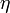$~\eta$, to mark the envelope's radial coordinate in our expressions. It is clear from other elements of his published derivation that Murphy understood this distinction but, as is explained more fully below, errors in his final model specifications may have resulted from not explicitly differentiating between this variable notation.

## Critique of Murphy's Model Characteristics

 Model Characteristics from Murphy's Table 3 Implications Model ξ = ζJ (Aξ) = ξJ A = ξJ / ζJ Δ = ln(Aξ)1 / 2 V1E V5F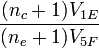$\frac{(n_c+1)V_{1E}}{(n_e+1)V_{5F}}$ 1 0.032678 10.0614 307.895 1.1544 3.5598E-04 1.1871E-04 1.000 2 0.8154 12.0083 14.727 1.2428 2.3212E-01 7.7302E-02 1.001 3 1.6598 20.4312 12.309 1.5085 1.1481 0.40720 0.940 4 2.6914 33.0249 12.2704 1.7486 6.5689 0.75371 2.905 5 2.7302 314.159 115.068 2.8750 7.2578 2.4193 1.000 6 3.1415 2.8675E+05 9.1278E+04 6.2832 3.3907E+04 2.9313E+04 0.386 NOTE: As is explained in an accompanying discussion, we suspect that the two numbers drawn from Murphy's Table 3 that are displayed here in a red font contain typographical errors.
 Some Model Characteristics (assuming μe / μc = 1) Model Specified Analytically Determined Here … and Constraint Implications†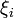$~\xi_i$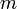$~m$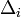$~\Delta_i$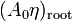$~(A_0\eta)_\mathrm{root}$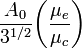$~\frac{A_0}{3^{1/2}} \biggl( \frac{\mu_e}{\mu_c} \biggr)$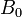$~B_0$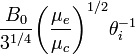$~\frac{B_0}{3^{1/4}} \biggl(\frac{\mu_e}{\mu_c}\biggr)^{1/2} \theta_i^{-1}$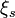$~\xi_s$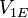$~V_{1E}$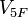$~V_{5F}$$\frac{(n_c+1)V_{1E}}{(n_e+1)V_{5F}}$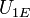$~U_{1E}$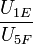$\frac{U_{1E}}{U_{5F}}$ 1 3.2678E-02 0 1.1544 10.0614 307.894 5.77929 4.39209 1.7392 3.5598E-04 1.1866E-4 1.000 2.9998 1.000 2 0.8154 0 1.2429 12.0101 14.729 1.25567 1.06865 36.356 0.23212 0.077372 1.000 2.8644 1.000 3 1.6598 0 1.4918 19.7585 11.9041 1.01206 1.28146 44.984 1.14812 0.38271 1.000 2.3995 1.000 4 2.0914 1 1.7328 31.9964 15.2990 0.87563 1.60398 35.0016 2.19913 0.73304 1.000 1.98894 1.000 5 2.7302 1 2.8749 100.00π 115.065 0.12408 0.64370 4.6539 7.25779 2.41926 1.000 1.02703 1.000 6 3.1415 2 6.283141 91268π 170.44223e2π 1.8966E-05 0.48862 3.141778 3.3907E+04 1.1302E+04 1.000 2.9106E-04 1.000 Corresponding Values Extracted Directly from Murphy's (1983) Table 3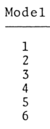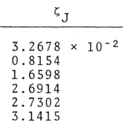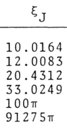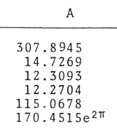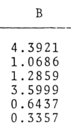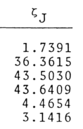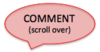†See an accompanying discussion for definitions of the functions,$~U_{1E}$,$~V_{1E}$,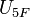$~U_{5F}$, and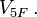$~V_{5F} \, .$

# Related Discussions

 © 2014 - 2021 by Joel E. Tohline |   H_Book Home   |   YouTube   | Appendices: | Equations | Variables | References | Ramblings | Images | myphys.lsu | ADS | Recommended citation:   Tohline, Joel E. (2021), The Structure, Stability, & Dynamics of Self-Gravitating Fluids, a (MediaWiki-based) Vistrails.org publication, https://www.vistrails.org/index.php/User:Tohline/citation